• 实对称矩阵对角化，有两篇论文，内含Matlab实现代码，在文章里的，可以直接写下来使用。测试过，还可以。
• A是一个实正交矩阵，给出了n维复向量组线性相关和线性无关的定义，证明了二阶正交矩阵对角化，以及某些三阶正交矩阵对角化
• 这里将哈密顿量写成二次型矩阵形式 ...因为费米子变换是幺正的，可以按照本征值对角化的方法处理。 和前面一样的方法，写成矩阵形式 这里和前面一样，写完后要乘一遍验证，由玻色子易关系，这里会出来一个常数...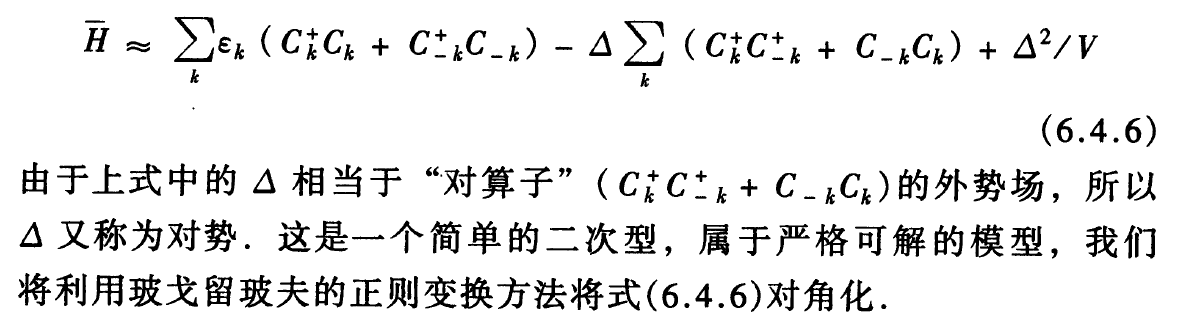这里将哈密顿量写成二次型矩阵形式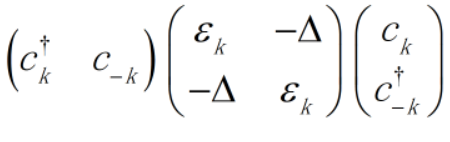注意，这个与一般的线性代数中的二次型不用，因为不满足交换律，需要手动乘一遍验证，这里写的很容易出错。

注意上面的等式是不对的，有一项是反过来的，需要用对易关系处理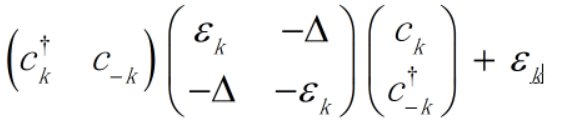因为费米子变换是幺正的，可以按照本征值对角化的方法处理。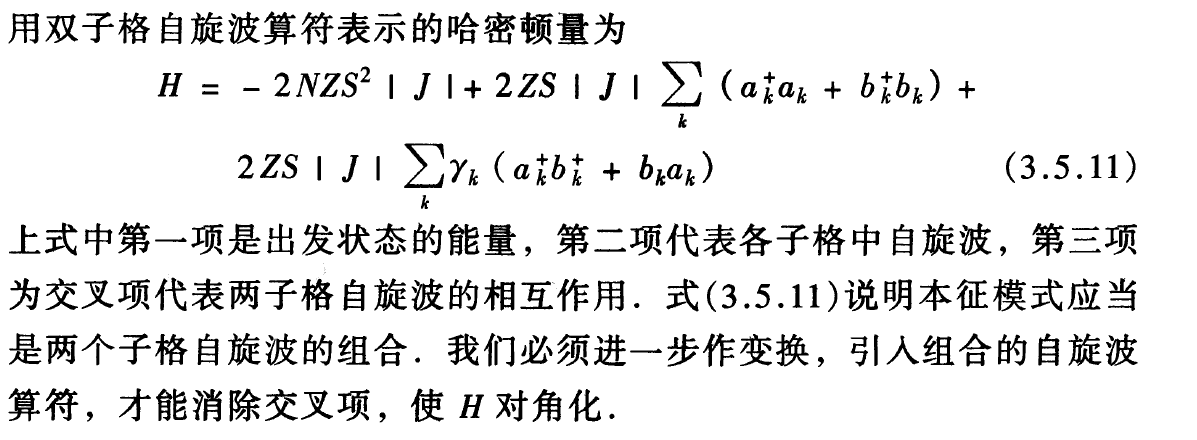和前面一样的方法，写成矩阵形式

这里和前面一样，写完后要乘一遍验证，由玻色子对易关系，这里会出来一个常数项。这里和费米子略有不同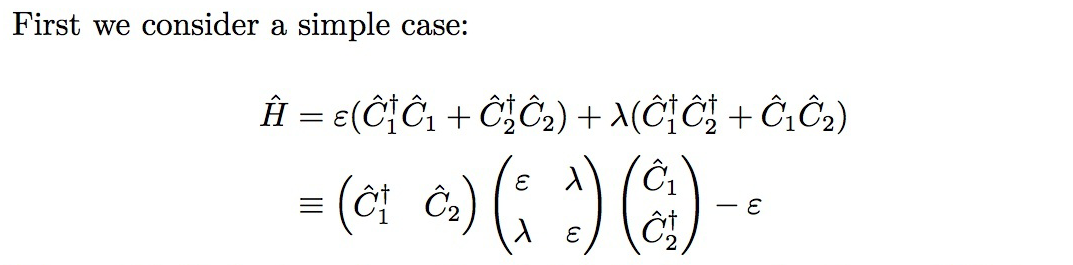然后下面是u-v变换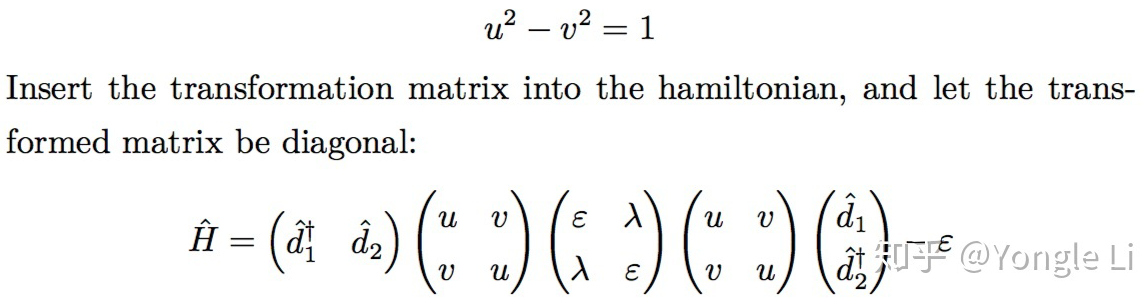玻色系统不能直接用矩阵对角化的方法处理，因为变换矩阵不是幺正矩阵，必须用矩阵乘法处理。

实际上处理的过程中，可以用到双曲三角函数来处理。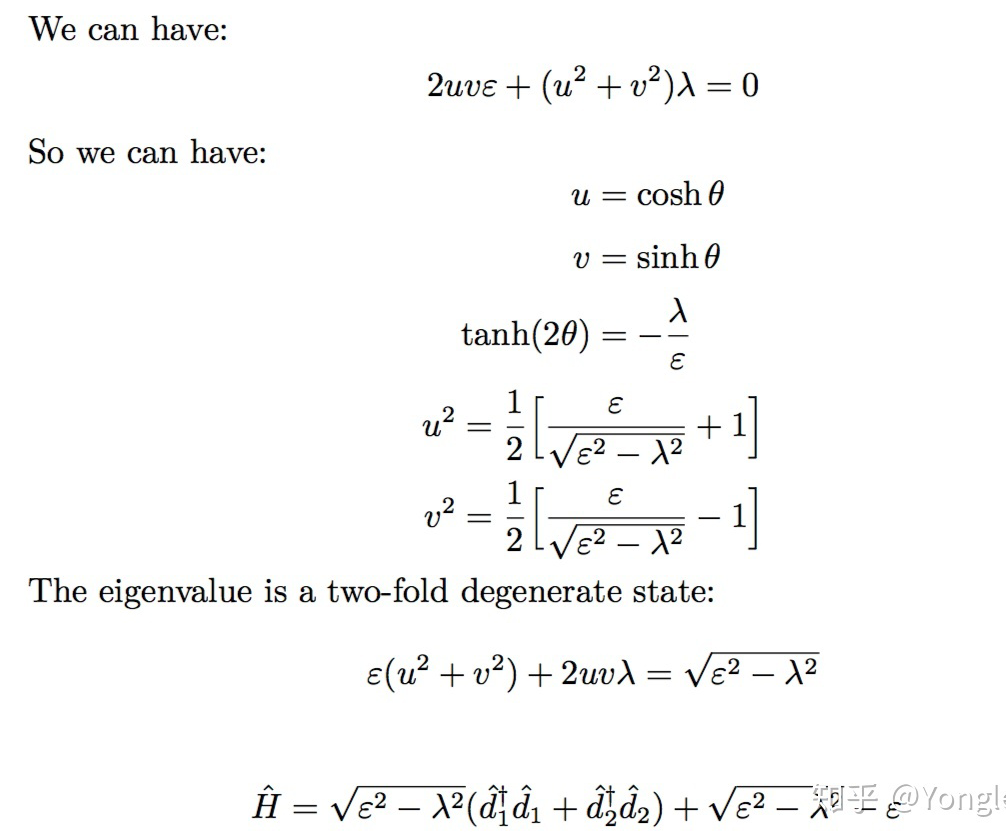总结，矩阵对角化方法先要利用将哈密顿量写成二次型矩阵的形式，这里不能直接写系数，需要先猜一个算符组合的形式，然后再乘一遍确认一下，需要用算符的对易关系处理一下。然后就可以将算符与系数分开来，直接处理矩阵就行了。

对于费米系统，由于变换是幺正的。所以可以直接用矩阵对角化的方法处理。这样不容易出错。

对于玻色系统，不是幺正变换，需要写出变换，矩阵，然后算矩阵乘法，再令非对角项为0。

相比起代数方法来看，矩阵方法更不容易出错。但手算的话计算量仍然很大。


展开全文• 文章目录矩阵和线性变换特征值与特征向量特征值求法奇异方阵相似矩阵对角化奇异值和奇异值分解 线性代数 向量,向量空间;矩阵, 线性变换; 特征值, 特征向量;奇异值, 奇异值分解 概率论与统计 随机事件;条件概率, 全...
文章目录矩阵和线性变换特征值与特征向量特征值求法奇异方阵相似矩阵对角化

线性代数
向量,向量空间;矩阵, 线性变换;
特征值, 特征向量;奇异值, 奇异值分解
概率论与统计
随机事件;条件概率, 全概率,贝叶斯概率;
统计量, 常见分布; 基本原理
最优化理论
极限, 导数;线性逼近, 泰勒展开;
凸函数, jensen不等式;最小二乘法; 梯度, 梯度下降

矩阵和线性变换
方阵能描述任意线性变换, 线性变换保留了直线和平行线, 但原点没用移动.
$\pmb v = \begin{bmatrix} x\\ y\\ z\\ \end{bmatrix} =\begin{bmatrix} x\\ 0\\ 0\\ \end{bmatrix}+\begin{bmatrix} 0\\ y\\ 0\\ \end{bmatrix}+\begin{bmatrix} 0\\ 0\\ z\\ \end{bmatrix}$
$\pmb v =\begin{bmatrix} x\\ y\\ z\\ \end{bmatrix}=x\times \begin{bmatrix} 1\\ 0\\ 0\\ \end{bmatrix}+y\times \begin{bmatrix} 0\\ 1\\ 0\\ \end{bmatrix}+z\times \begin{bmatrix} 0\\ 0\\ 1\\ \end{bmatrix}$
向量的每一个坐标都表明了平行于相应坐标轴的有向位移.
如果把矩阵的行解释为坐标系的基向量，那么乘以该矩阵就相当于执行了一次坐标转换，如果$aM=b$，我们就可以说，$M将a$转换到$b$。
从这点看，术语“转换”和“乘法”是等价的。
坦率地说，矩阵并不神秘，它只是用一种紧凑的方式来表达坐标转换所需的数学运算。进一步，用线性代数操作矩阵，是一种进行简单转换或导出更复杂转换的简便方法.
我们可以不依赖于坐标系而沿任意方向进行缩放，设$\vec{n}$为平行于缩放方向的单位向量，k为缩放因子,缩放沿穿过原点并平行于$\vec{n}$的直线（2D中）或平面（3D中）进行。
$\vec{v} = \vec{v}_{||}+\vec{v}_{⊥}$
$\vec{v}_{||}=(\vec{v}\cdot \vec{n})\vec{n}$
$\vec{v}_{⊥}=\vec{v}-\vec{v}_{||}=\vec{v}-(\vec{v}\cdot \vec{n})\vec{n}$
$\vec{v}^{'}=\vec{v}_{||}^{'}+\vec{v}_{⊥}^{'}$
$\vec{v}_{⊥}^{'}=\vec{v}_{⊥}=\vec{v}-(\vec{v}\cdot\vec{n})\vec{n}$
$\vec{v}_{||}^{'}=k\cdot\vec{v}_{||}=k\cdot(\vec{v}\cdot\vec{n})\vec{n}$
$\vec{v}^{'}=\vec{v}_{⊥}=\vec{v}-(\vec{v}\cdot\vec{n})\vec{n}+k\cdot(\vec{v}\cdot\vec{n})\vec{n}=\vec{v}+(k-1)(\vec{n}\cdot\vec{v})\vec{n}$
（待补充）
特征值与特征向量
定义:$A $n阶方阵, 对于$\lambda$, 存在非零向量$\vec v$, 使得$\pmb A\vec v=\lambda\vec v$,则:$\lambda$就叫特征值, $\vec v$就叫对应$\lambda$的特征向量
特征值可以为0, 特征向量不能为0
$\pmb A\vec x =\lambda \vec x ~~~~ x\not= 0$
↓
$(\pmb A-\lambda \pmb E)\vec x = 0$
↓
$|\pmb A-\lambda\pmb E|=0$
其中$\lambda$和$x$为我们需要求得值

$\pmb Ax$代表向量的线性变换,$\lambda x$代表向量拉伸变换
特征向量的含义就在于使得哪些向量只发生拉伸变换
而特征值用于衡量相应的拉伸系数
特征值就是运动的速度, 特征向量就是运动的方向

注:只有方阵才能计算特征值和特征向量

例:
$\pmb A=\begin{bmatrix} 4&0\\ 3&-5\\ \end{bmatrix}$
求特征值:
$|\pmb A-\lambda \pmb E|$
$=\begin{bmatrix} 4-\lambda&0\ 3&-5-\lambda \end{bmatrix}$
$=(4-\lambda)(-5-\lambda)=0$
得:$\lambda_{1}=-5, \lambda_{2}=4$
针对特征值$\lambda_{1}=-5$, 计算特征向量 $\pmb X_{1}$
$\begin{bmatrix} 9&0\\ 3&0\\ \end{bmatrix}\cdot \vec x=0~~~\pmb X_{1}=\begin{bmatrix}0\\1\\\end{bmatrix}$
针对特征值$\lambda_{2}=4$, 计算特征向量$\pmb X_{2}$
$\begin{bmatrix}0&0\\3&-9\\\end{bmatrix}\cdot\vec x=0~~~\pmb X_{2}=\begin{bmatrix}3\\1\\\end{bmatrix}$
例:
$\pmb A=\begin{bmatrix}4&-2\\3&-1\\\end{bmatrix}$
求特征值:
$|\pmb A-\lambda \vec x|=\begin{bmatrix}4-\lambda&-2\\3&-1-\lambda\end{bmatrix}=(4-\lambda)(-1-\lambda)+6=0$
得:$\lambda_{1}=1, \lambda_{2}=2$
针对特征值$\lambda_{1}=1$, 计算特征向量$\pmb X_{1}$
$\begin{bmatrix}3&-2\\3&-2\end{bmatrix}\cdot\vec x=0~~~\pmb X_{1}=\begin{bmatrix}2\\3\\\end{bmatrix}$
针对特征值$\lambda_{2}=2$, 计算特征向量$\pmb X_{2}$
$\begin{bmatrix}2&-2\\3&-3\\\end{bmatrix}\cdot \vec x=0~~~\pmb X_{2}=\begin{bmatrix}1\\1\\\end{bmatrix}$
另一种计算方式,首先将$\vec x$表示成特征向量$\begin{bmatrix}1\\1\\\end{bmatrix}$和$\begin{bmatrix}2\\3\\\end{bmatrix}$的线性组合,即:
$\vec x=\begin{bmatrix}1\\2\\\end{bmatrix}=-1\cdot\begin{bmatrix}1\\1\\\end{bmatrix}+1\cdot\begin{bmatrix}2\\3\\\end{bmatrix}$
然后,将特征值与对应系数(特征值)相乘, 得到:
$\vec y = -1\cdot2\cdot\begin{bmatrix}1\\1\\\end{bmatrix}+1\cdot1\cdot\begin{bmatrix}2\\3\\\end{bmatrix}=\begin{bmatrix}0\\1\\\end{bmatrix}$
这与$\vec y=\pmb A\vec x=\begin{bmatrix}0\\1\\\end{bmatrix}$相同,表示$\pmb A$对向量$\vec x$的线性变换相当于$\pmb A$的特征值和特征向量与$\vec x$的线性组合, 可以说在线性变换时, 矩阵的特征值和特征向量可以代表矩阵.
矩阵所充当的映射, 实际上就是对特征向量的缩放, 每个特征向量的缩放程度就是特征值.
将$\vec x$向量表示特征向量的线性组合(相当于以特征向量为基),得到相应的特征向量的权重.然后,每个权重与特征值相乘, 就是这个映射最本质的缩放操作.

特征值求法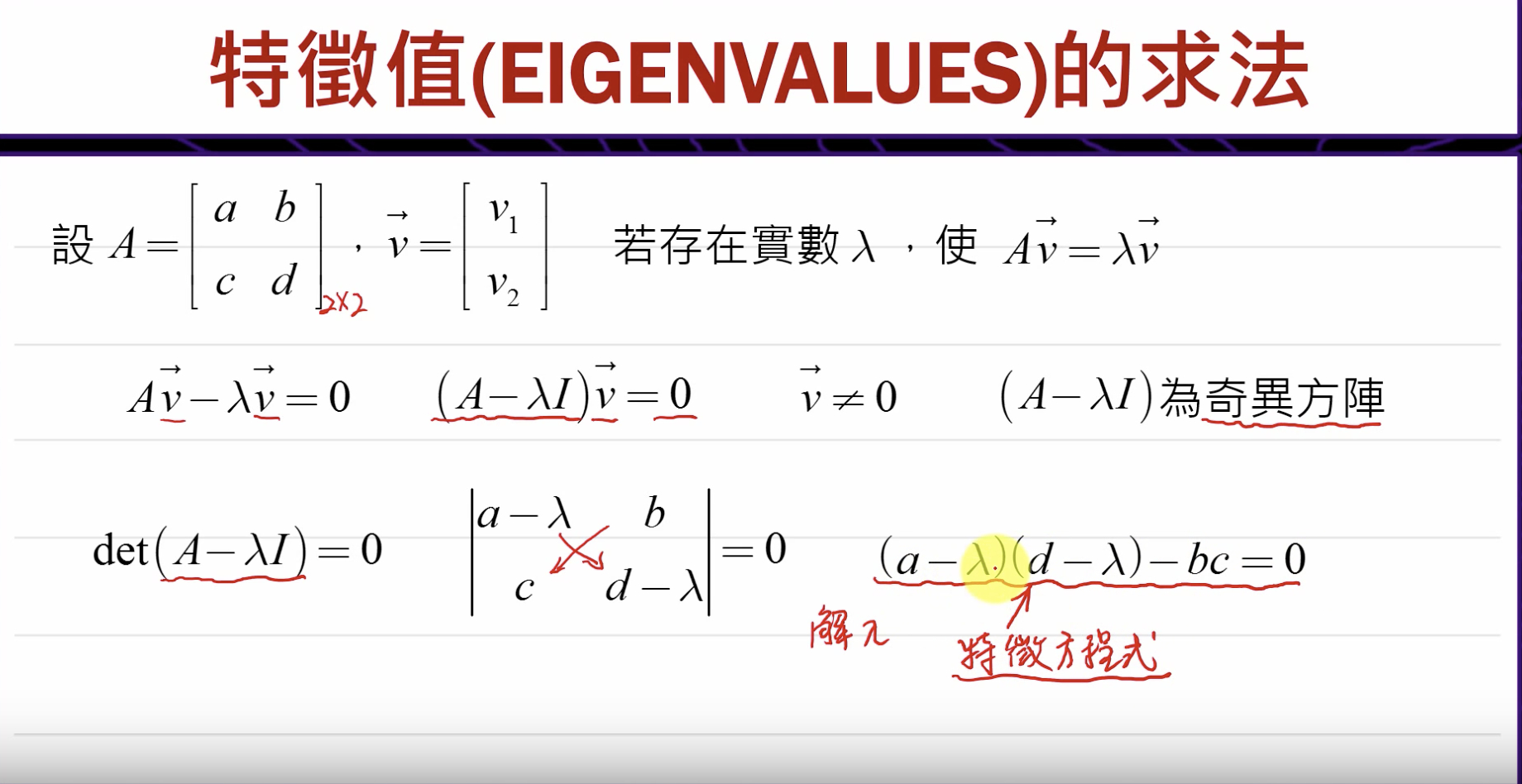奇异方阵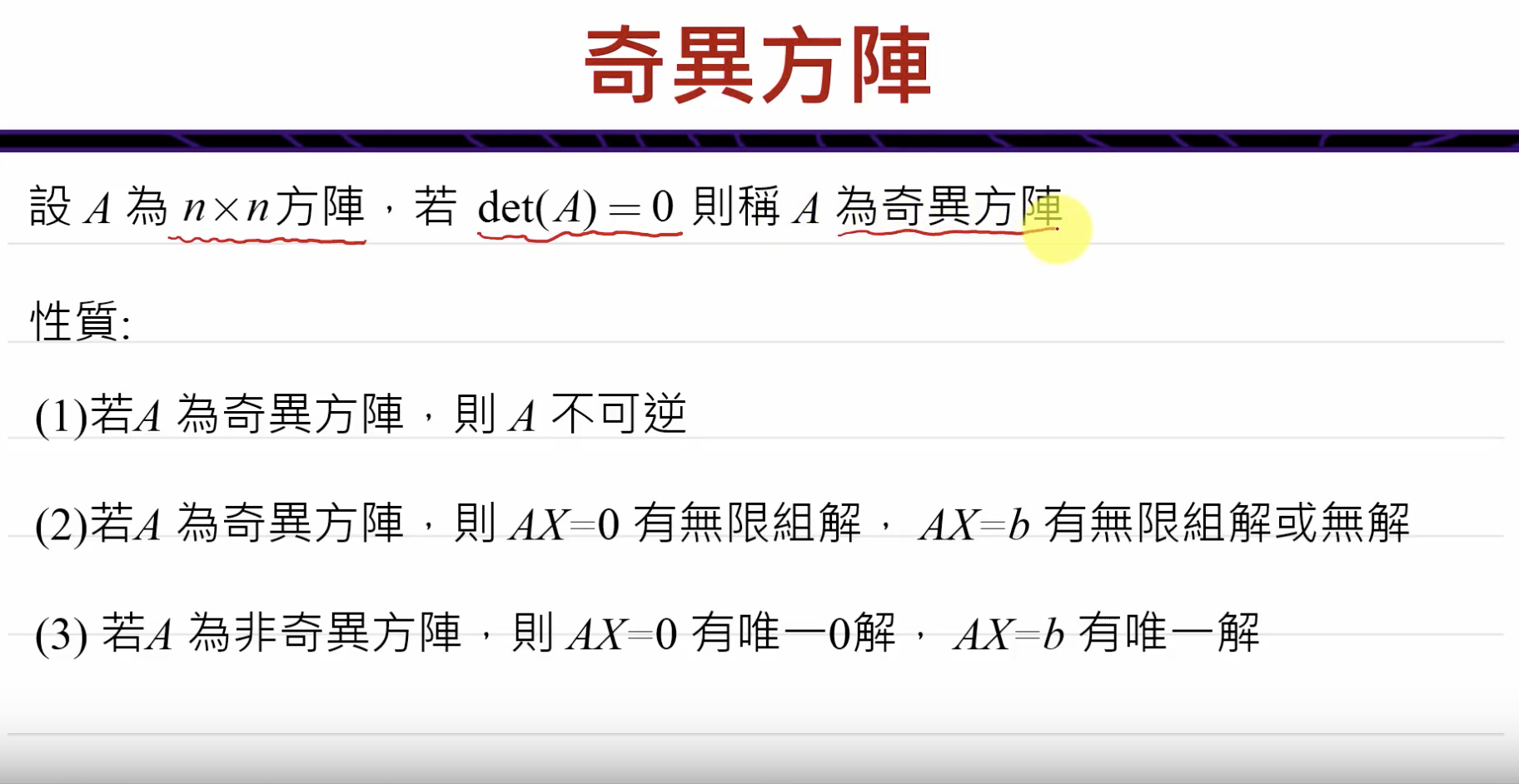相似矩阵
定义:若$\pmb A$与$\pmb B$均为n阶方阵, 若存在一个可逆矩阵$\pmb P$, 使$\pmb P^{-1}\cdot A \cdot \pmb P=B$, 则称$\pmb A$与$\pmb B$相似
对角化

定义及证明
定义:假设一个$n\times n$阶的方阵$\pmb A$,有 $n$个线性无关的特征向量$v_1, v_2,\cdots, v_n$, 所有的特征向量组成特征向量矩阵$\pmb S$, 则有$\pmb S^{-1}\pmb A\pmb S=\Lambda$, 其中$\Lambda$为由$\pmb S$对应的特征值组成的对角矩阵, 即:
$\pmb S^{-1}\pmb A\pmb S=\Lambda=\begin{bmatrix} \lambda_1\\ &\ddots\\ &&\lambda_n \end{bmatrix}$
证明:
$\pmb A\pmb S=\pmb A\begin{bmatrix}v_1&v_2&v_3&\cdots&v_n\end{bmatrix}=\begin{bmatrix}\lambda_1v_1&\lambda_2v_2&\lambda_3v_3&\cdots&\lambda_nv_n\end{bmatrix}=\pmb S\begin{bmatrix}\lambda_1\\&\ddots\\&&\lambda_n\end{bmatrix}=\pmb S\pmb{\Lambda}$
$\pmb S^{-1}\pmb A\pmb S=\pmb S^{-1}\pmb S\pmb{\Lambda}=\pmb{\Lambda}$
$\pmb A=\pmb S\pmb {\Lambda}\pmb S^{-1}$(矩阵对角化)

例:
$\pmb A=\begin{bmatrix} -3&2\\-10&6\\\end{bmatrix}$
对角化$\pmb A$.
解:
$\pmb A-\lambda\pmb E=\begin{bmatrix}-3-\lambda&2\\-10&6-\lambda\end{bmatrix}$
$(-3-\lambda)(6-\lambda)+20=0$
$\lambda_1=1, ~~~\lambda_2=2$
对应$\lambda_1$的特征向量$v_1$:
$\begin{bmatrix}-4&2\\-10&5\end{bmatrix}\cdot v_1=0, ~~~v_1=\begin{bmatrix}1\\2\end{bmatrix}$
对应$\lambda_2$的特征向量$v_2$:
$\begin{bmatrix}-5&2\\-10&4\end{bmatrix}\cdot v_2=0, ~~~v_2=\begin{bmatrix}2\\5\end{bmatrix}$
$\pmb P=\begin{bmatrix}\vec v_1&\vec v_2\end{bmatrix}=\begin{bmatrix}1&2\\2&5\\\end{bmatrix}$
$\pmb P^{-1}=\begin{bmatrix}5&-2\\-3&1\end{bmatrix}$(二阶时, 主对角线对换, 负对角线变号/det( P ))


展开全文机器学习 深度学习 线性代数
• 矩阵对角化一节略有琐碎，对于如何判断矩阵对角化——代数重数和几何重数相等，笔者已经在第五篇线代笔记中给出了相应的定义以及数学层面的证明。在第六篇笔记中笔者将对矩阵对角化给出粗略的梳理，涉及定义、矩阵...

文章转载请注明出处，加上原文地址链接，谢谢！https://blog.csdn.net/weixin_46959681/article/details/106135582

文章目录前言矩阵对角化的定义对角化应用例题方面的分析｜经典的斐波那契数列求通项｜矩阵方幂的求取——MIT线代习题课 T23｜马尔可夫矩阵计算粒子跳跃——MIT线代习题课T25矩阵对角化的结论矩阵的指数函数形式｜矩阵的指数函数性质特征值在微分方程中的应用二阶常系数线性微分方程｜微分方程 $\mathsf{\frac{du}{dt}=Au}$ 的解的稳定性的分析及归纳图参考资料文章更新时间记录

前言
矩阵对角化一节略有琐碎，对于如何判断矩阵对角化——代数重数和几何重数相等，笔者已经在第五篇线代笔记中给出了相应的定义以及数学层面的证明。在第六篇笔记中笔者将对矩阵的对角化给出粗略的梳理，涉及定义、矩阵对角化相应的例题（经典斐波那契数里的通项求解、MIT线代习题公开课T23求矩阵的方幂、MIT线代习题公开课T25粒子的跳跃）以及相应的结论。对于对角化一节会粗粗略过，该篇重点回放在第二部分——特征值在微分方程中的应用。对上一篇笔记有印象的朋友肯定会记得，笔者在已经给出一个常系数微分方程的例题。在这里笔者会涉及更多的东西，如以微分方程的角度探讨矩阵对角化的情形，从指数函数的层面推出矩阵 $e^{At}$ 的导数公式，最后给出微分方程解的稳定性分析以及归纳图。下面我们正式开始。

矩阵对角化的定义
定义 如果n阶矩阵A有n个线性无关的特征向量 $\mathsf{(v_1,\cdots,v_n)},$ 令 $\mathsf{S=(v_1,v_2,\cdots,v_n)},$ 则有$\mathsf{AS=S\begin{pmatrix}\lambda_1& & \\ &\lambda_2& \\ & & \ddots& \\ & & &\lambda_n\\\end{pmatrix}=S\Lambda}$故 $\mathsf{S^{-1}AS=\Lambda}$ 为对角阵。此时称矩阵$\mathsf{A}$可对角化。

光有定义印象还不够深刻，下面笔者引用经典的斐波那契数列的通项和两道MIT线代习题课内的两道题帮助大家加深印象。对于已经熟悉的朋友快速过一篇例题，略有生涩的朋友可以点击链接，跳转观看讲解视频。

对角化应用例题方面的分析
｜经典的斐波那契数列求通项
题 求斐波那契数列 $\mathsf{0,1,1,2,3,5,8\cdots}$ 的通项？
解： 令 $\mathsf{u_k=\begin{pmatrix}F_{k+1}\\F_k\\\end{pmatrix}},$ 推敲数列前后两项可得  $\mathsf{\begin{cases}F_{k+2}=F_{k+1}+F_k\\F_{k+1}=F_{k+1}\\\end{cases}}$则 $\mathsf{u_{k+1}}$ 矩阵的形式为 $\mathsf{u_{k+1}=\begin{pmatrix}1&1\\1&0\\\end{pmatrix}u_k\longrightarrow Au_k=u_{k+1}}$ 故有 $\mathsf{det(A-\lambda I)=\begin{vmatrix}{1-\lambda}&{1}\\{1}&{-\lambda}\\\end{vmatrix}=\lambda^2-\lambda-1=0}$ 解得 	$\mathsf{\lambda_1=\frac{1+\sqrt5}{2}，\lambda_2=\frac{1-\sqrt5}{2}\lt 0}$ 故通项 $\mathsf{F_n=C_1(\frac{1+\sqrt5}{2})^n+C_2(\frac{1-\sqrt5}{2})^n}$

因通项的后半段中 $\mathsf{\frac{1-\sqrt5}{2}\lt0},$ 故无穷后趋向于零。这里有一个非常深刻的地方，为什么一个全部是有理数的数列，其数列的通项推导出来会有无理数被包含在内呢？ 这里笔者对于数论并没有研究，无法深入探讨，阅读的朋友可以自行挖掘。

｜矩阵方幂的求取——MIT线代习题课 T23

教学视频链接 https://www.bilibili.com/video/BV19b411E7ue?p=23

题 对矩阵 $\mathsf{C=\begin{pmatrix}{2b-a}&{a-b}\\{2b-2a}&{2a-b}\\\end{pmatrix}},$ 并计算当 $\mathsf{a=b=-1}$ 时，求取 $\mathsf{C^{100}}$。
解：
$\mathsf{det(C-\lambda I)=det\begin{vmatrix}{(2b-a)-\lambda}&{a-b}\\{2b-2a}& {(2a-b)-\lambda}\\\end{vmatrix}=(\lambda-a)(\lambda-b)=0}$  解,得 $\mathsf{\lambda_1=a,\lambda_2=b}$
对应特征值 $\mathsf{\lambda_1=a}$ 特征向量 $\mathsf{v_1}$ 的计算：$\mathsf{(c-aI)v_1=\begin{pmatrix}{2b-2a}&{a-b}\\{2b-2a}&{a-b}\\\end{pmatrix}v_1=0 \rightarrow v_1=\begin{pmatrix}1\\2\\\end{pmatrix}}$
对应特征值 $\mathsf{\lambda_2=b}$ 特征向量 $\mathsf{v_2}$ 的计算：
$\mathsf{(c-bI)v_2=\begin{pmatrix}{b-a}&{a-b}\\{2b-2a}&{2a-2b}\\\end{pmatrix}v_2=0 \rightarrow v_2=\begin{pmatrix}1\\1\\\end{pmatrix}}$
故特征向量矩阵 $\mathsf{S=\begin{pmatrix}1&1\\2&1\\\end{pmatrix}},$ 其逆矩阵 $\mathsf{S^{-1}=\frac{1}{det S}(S_{ij})^T=\begin{pmatrix}-1&1\\2&-1\\\end{pmatrix}},$
则有  $\mathsf{C=S^{-1}\Lambda S=\begin{pmatrix}1&1\\2&1\\\end{pmatrix}\begin{pmatrix}a& \\ &b\\\end{pmatrix}\begin{pmatrix}-1&1\\2&-1\\\end{pmatrix}}$
所以 $\mathsf{C^k=S^{-1}\Lambda^K S=\begin{pmatrix}1&1\\2&1\\\end{pmatrix}\begin{pmatrix}a^K& \\ &b^K\\\end{pmatrix}\begin{pmatrix}-1&1\\2&-1\\\end{pmatrix}=\begin{pmatrix}{2b^k-a^k}&{a^K-b^k}\\{2b^K-2a^K}&2a^K-b^K\\\end{pmatrix}}$
故$\mathsf{C^{100}=S\Lambda^{100}S^{-1}=\begin{pmatrix}1&0\\0&1\\\end{pmatrix}=I}$

想必仔细阅读下来的朋友可以理解矩阵的对角化对于求幂次矩阵的的强大威力。注意 $(S_{ij})^T$ 是代数余子式矩阵的转置矩阵。

｜马尔可夫矩阵计算粒子跳跃——MIT线代习题课T25

教学视频链接 https://www.bilibili.com/video/BV19b411E7ue?p=25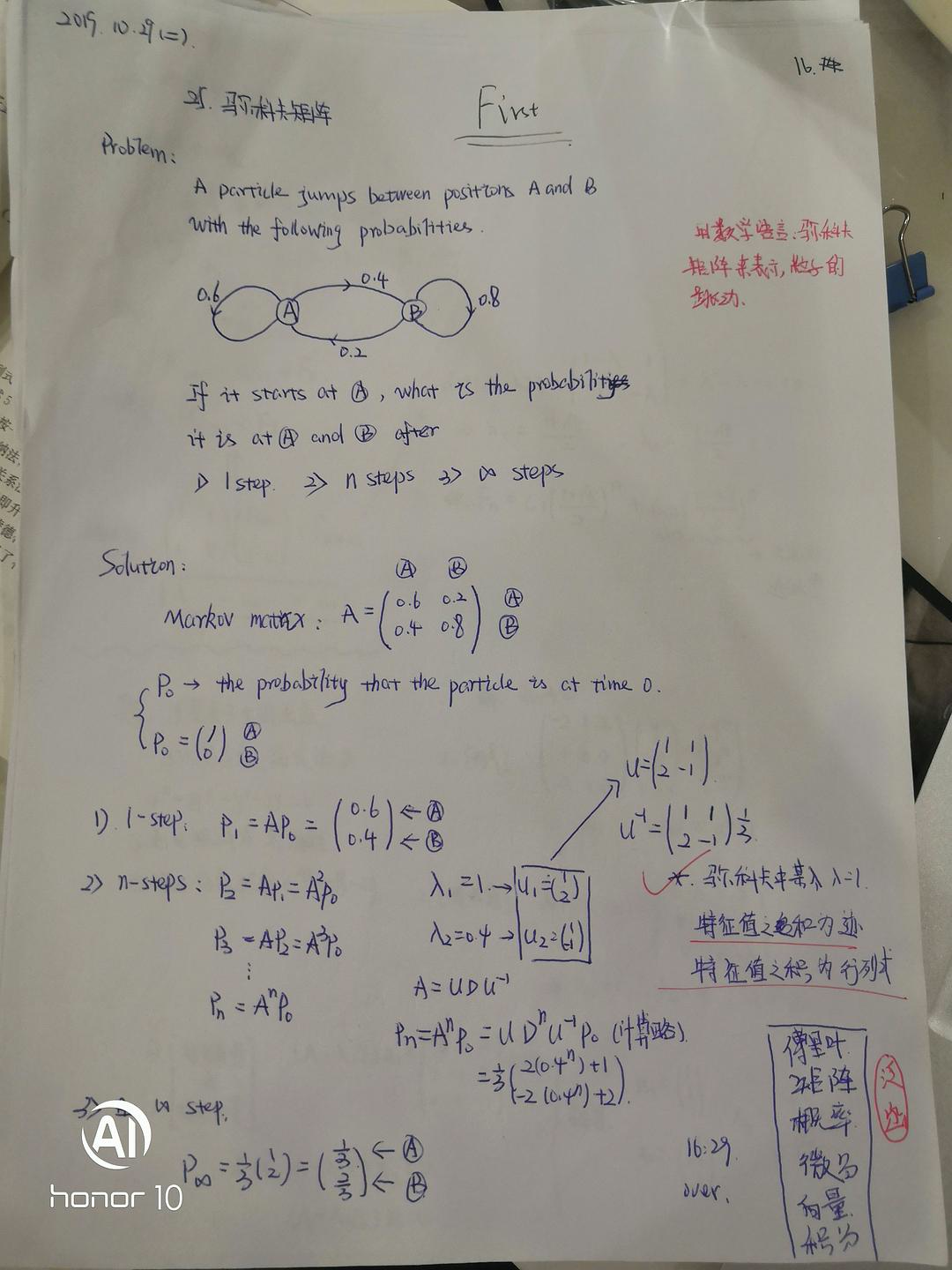矩阵对角化的结论

矩阵A可对角化，值存在可逆矩阵S，使 $\mathsf{S^{-1}AS=\Lambda}$ 为对角阵且$\mathsf{A^k=S\Lambda^kS^{-1}}$；
$n\times n$ 矩阵对角化$\longleftrightarrow$A有n个线性无关的特征向量；
$n\times n$ 复矩阵A有n个互异特征值，则A可对角化；
$n\times n$ 复矩阵A可对角化$\longleftrightarrow$任意特征值的几何重数等于代数重数；
差分方程$\mathsf{u_{k+1}=Au_k}$的解为$\mathsf{u_k=A^ku_0=S\Lambda^kS^{-1}u_0=c_1\lambda_1^kx_1+\cdots+c_n\lambda_n^kx_n},$其中$\mathsf{u_0=c_1x_1+\cdots+c_nx_n,Ax_i=\lambda_ix_i(1\le i \le n)}.$

矩阵的指数函数形式
对于$\mathsf{e^x=\displaystyle\sum_{n=0}^{\infty}=1+x+\frac{x^2}{2!}\cdots+\frac{x^n}{n!}+\cdots}$ 推出 $\mathsf{e^{At}=I+At+\frac{(At)^2}{2!}\cdots+\frac{(At)^n}{n!}+\cdots}$ 推出 $\mathsf{\frac{d(e^{At})}{dt}=A+A^2t+\frac{A^3t^2}{2!}+\cdots=A(I+At+\frac{(At)^2}{2!}+\cdots)=Ae^{At}}$ 推出 $\mathsf{u(t)=e^{At}u(0)为 \frac{du}{dt}=Au的解}$

｜矩阵的指数函数性质

如果 $\mathsf{\Lambda=\begin{pmatrix}\lambda_1& & \\ &\ddots& \\ & &\lambda_n\\\end{pmatrix}},$ 则 $\mathsf{e^{\Lambda t}=\begin{pmatrix}e^{\lambda_1t}& & \\ &\ddots& \\ & &e^{\lambda_nt}\\\end{pmatrix}=\begin{pmatrix}1+\lambda_1t+\frac{(\lambda_1t)^2}{2!}+\cdots+\frac{(\lambda_1t)^n}{n!}+\cdots\\ &\ddots& \\ & &1+\lambda_nt+\frac{(\lambda_nt)^2}{2!}+\cdots+\frac{(\lambda_nt)^n}{n!}\cdots\\\end{pmatrix}}$
如果 $\mathsf{AB=BA},$ 则 $\mathsf{e^{A+b}=e^A*e^B},$ 特别有 $\mathsf{(e^A)^{-1}=e^{-A}}$ 且$\mathsf{e^{A+B}=I+(A+B)+\frac{(A+B)^2}{2!}+\cdots}$ $\mathsf{e^{AB}=(I+A+\frac{A^2}{2!}+\cdots)(I+B+\frac{B^2}{2!}+\cdots)}$
如果存在可逆矩阵P使$\mathsf{A=PBP^{-1}},$ 则 $\mathsf{e^{At}=e^{PBP^{-1}t}}$
且$\mathsf{e^{At}=e^{PBP^{-1}T}=I+PBP^{-1}t+\frac{(PBP^{-1})^2}{2!}+\cdots=P(I+Bt+\frac{(Bt)^2}{2!}+\cdots)P^{-1}}$

特征值在微分方程中的应用
一般地，如果$\mathsf{A=S\Lambda S^{-1}}$可对角化，类似于求解$\mathsf{u_k=A^ku_0},$ 有  $\mathsf{\frac{du}{dt}=Au=S\Lambda S^{-1}u \Longleftrightarrow \frac{d(S^{-1}u)}{dt}=\Lambda(S^{-1}u)},$ 即$\mathsf{S^{-1}u=\begin{pmatrix}e^{\lambda_1t}C_1\\e^{\lambda2t}C_2\\\vdots\\e^{\lambda_nt}C_n\\\end{pmatrix}\rightarrow u(t)=C_1e^{\lambda_1t}x_1+\cdots+C_ne^{\lambda_n}tx_n}$ 同时，对于 $\mathsf{\frac{du}{dt}=A_{n\times n}u}$ 的解集是一个n维向量空间。如果A可对角化，则 $\mathsf{\frac{du}{dt}=Au}$ 的解空间有一组基$\mathsf{\lbrace e^{\lambda_1t}x_1,e^{\lambda_2t}x_2\cdots,e^{\lambda_nt}x_n\rbrace}$

上述给出了微分方程层面出发的对角化，下面我们来看看特征值与二阶微分方程关系。

二阶常系数线性微分方程
设 $\mathsf{y=e^{\lambda t}}$  是二阶微分方程 $\mathsf{y''+ay'+by=0}$ 的解，则有 $\mathsf{\lambda^2+a\lambda+b=0}$。如果解 $\mathsf{\lambda_1\neq \lambda_2},$ 则 $\mathsf{y_1=e^{\lambda_1t},y=e^{\lambda_2t}}$ 为两个线性无关的解，以下：

若 $\mathsf{\lambda_1,\lambda_2}$ 为实数，则方程的通解为 $\mathsf{y=C_1e^{\lambda_1t}+C_2e^{\lambda_2t}};$
若 $\mathsf{\lambda_1,\lambda_2}$ 为共轭复数，即
$\mathsf{\left \{ \begin{array}{c} \lambda_1=\alpha+i\beta\\ \lambda_2=\alpha-i\beta\\ \end{array} \right.}$

方程组的解为
$\mathsf{y=e^{\alpha t}(C_1\cos\beta t+C_2\sin\beta t)}$
欧拉公式：
$\mathsf{\frac{e^{(\alpha+i\beta)}+e^{(\alpha-i\beta)}}{2}=e^{\alpha t}\cos\beta t}$ $\mathsf{\frac{e^{(\alpha+i\beta)}-e^{(\alpha-i\beta)}}{2}=e^{\alpha t}\sin\beta t}$

博客修改失误，图片彻底消失。😳

｜微分方程 $\mathsf{\frac{du}{dt}=Au}$ 的解的稳定性的分析及归纳图
$\mathsf{t\longrightarrow \infty}$ 时，$\mathsf{A}$ 的特征值决定解 $u(t)$ 的稳定性。若 $\mathsf{A}$ 可对角化，则 $\mathsf{\frac{du}{dt}=Au}$ 有通解，$\mathsf{u(t)=(x_1,\cdots,x_n)\begin{pmatrix}e^{\lambda_1t}& & \\\ & \ddots& \\ & &e^{\lambda_nt}\\\end{pmatrix}\begin{pmatrix}C_1\\\vdots\\C_n\\\end{pmatrix}}$

若所有$\mathsf{Re\lambda_i \lt 0}$，则 $\mathsf{e^{At}\rightarrow0}$ 解时稳定的；
若所有$\mathsf{Re\lambda_i \le 0}$，则 $\mathsf{e^{At}}$ 有界，解是中性的；
若至少有一个特征值满足 $\mathsf{Re\gt 0}$ ，则 $e^{At}$ 无界，解不稳定。

对于解的稳定性分析，我们可以归纳成下面的一张图片：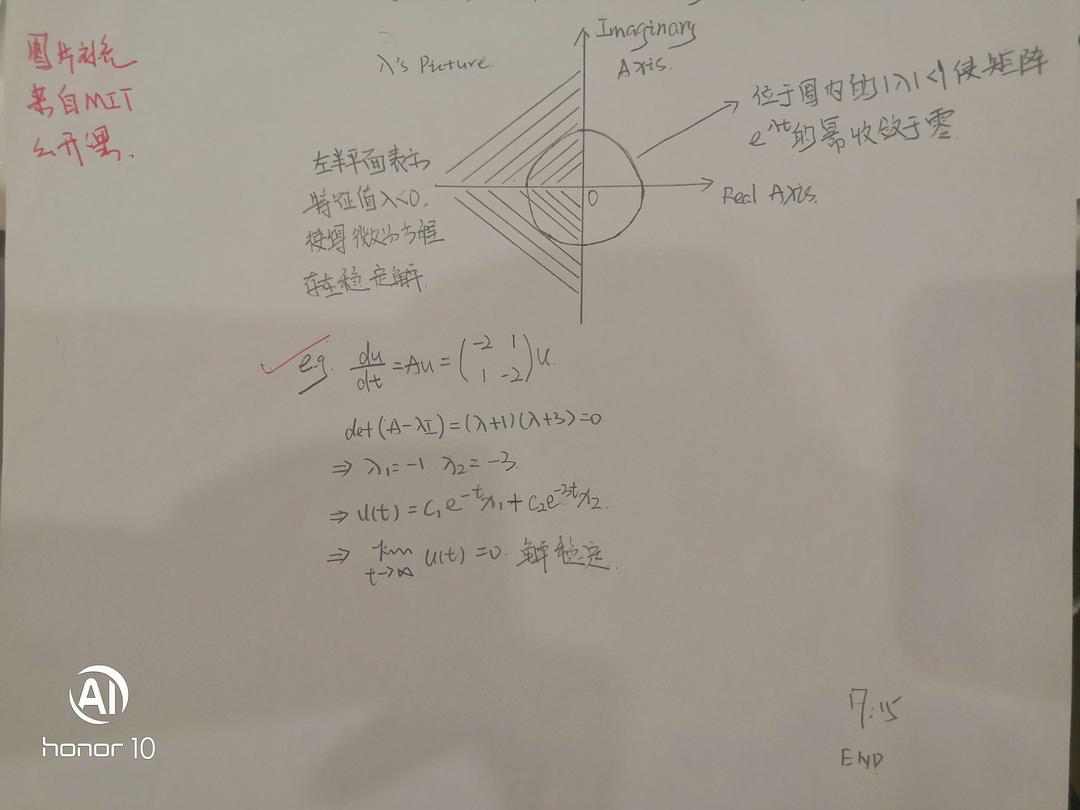参考资料

笔者个人清华大学线性代数公开课笔记
笔者个人MIT线性代数公开课笔记
笔者个人MIT线性代数习题公开课笔记

文章更新时间记录

文章版式微微调整。「2020.6.1 21:22」
文章版式巨大调整。「2021.3.5 18:35」

P.S. 笔者在该篇笔记的归纳整理的过程中感觉异常的不顺畅，相信通读下来的朋友应该或多或少感觉该篇有强行总结的感觉——尤其是第二部分特征值与微分方程的关系。在行文中这种感觉始终摆脱不得，最后文章成形颇有四不像的感觉，勉强看看吧。耕耘不易，有得请点赞，谢谢🙏！


展开全文线性代数 机器学习
• 矩阵对角化：AS = S（讲AS展开可以推导出这个公式） 上式两边的左边同时乘以S-1，得出S-1AS = 。这就是方阵的对角化公式 上式两边的右边同时乘以S-1，得出A = SS-1，这就是矩阵的句对话分解。   如果A的特征值...
特征向量矩阵S，由矩阵A的所有线性无关的特征向量按列排列组成的矩阵。

特征值矩阵，有矩阵A的所有特征值放在对角线位置组成的对角矩阵。

矩阵对角化：AS = S（讲AS展开可以推导出这个公式）

上式两边的左边同时乘以S-1，得出S-1AS = 。这就是方阵的对角化公式

上式两边的右边同时乘以S-1，得出A = SS-1，这就是矩阵的句对话分解。

如果A的特征值都不相同，则A存在n个线性无关的特征向量，并且可对角化。

存在n个线性无关的特征向量是可以矩阵可以对角化的前提。

如果A存在重复的特征值，可能存在n个线性无关的特征向量，也可能不存在，要视具体情况而定。

求解一阶差分方程组，给定向量u（0） 求解u（k+1） = Au（k）

u1 = Au0

u2 =A*Au0.

可知u（k） = A（k）u（0）。这就是一阶差分方程的解。

利用矩阵的幂可以求解斐波那契数列的代数表达式。

f（k+2） = f（k+1）+f（k） 这是斐波那契数列的递归式，是一个二阶差分方程。

f（k+1） = f（k+1） 这个式子与上面的式子组成一个线性系统。由此可以求出代数表达式。


展开全文• n），且保持二阶残差范数最小： Ax=bMin∥Ax−b∥2 Ax=b\\ Min\parallel Ax-b \parallel_{2} Ax=bMin∥Ax−b∥2​ 对于传统的最小二乘问题，可以直接求出方程的解，但是对于大型稀疏矩阵，往往是病态问题（超定方程...
• (来自质数) 设$A,B$ 都是实数域上的两个二阶方阵, 且 $AB=BA$....证明: (来自 torsor) 因为 $A,B$ 可交换, 所以在复数域上它们可以同时上角化. 这一结论可以参考复旦高代教材第六章总复习题18...
• 转置矩阵： 将矩阵的行列互换得到的新矩阵称为转置矩阵，转置矩阵的行列式不变。 例如， ， 。 如果阶方阵和它的转置相等 ，即，则称矩阵为对称矩阵。 如果，则称矩阵为反对称矩阵。 正交矩阵： 如果：AAT=E...
• 第二十二课时：对角化和A的幂 Ax=λx,特征值、特征向量的应用以及为什么需要特征值和特征向量。 对角化 S-1AS=Λ 假设A有n个线性无关的特征向量（大前提），将他们按列组成矩阵S，S为特征向量矩阵，那么S-斐波拉契数列 差分方程
• ## 对角矩阵_分块矩阵

千次阅读 2015-09-24 15:20:00
1.对角矩阵 　不在主对角线上的元素全部为0的n阶方阵，称为对角矩阵. 2.分块矩阵对角阵 　 转载于:https://www.cnblogs.com/chaoren399/p/4835448.html
• 矩阵A无逆矩阵,则称A为奇异矩阵。若A有逆矩阵,则称A是非奇异矩阵,简称非异阵 N阶行列式的超平行多面体的几何图形是由行(或列)向量张成的，而且这个n维超平行多面体与一个n维超长方体等体积。 一、代数意义 ...
• 矩阵对矩阵的求导采用了向量的思路，常应用于二阶方法求解优化问题。 首先来琢磨一下定义。矩阵对矩阵的导数，需要什么样的定义？ 第一，矩阵F(p×q)F(p×q)F(p×q)对矩阵X(m×n)X(m×n)X(m×n...
• 实正交矩阵的行列式只能为1或-1，为1的是旋转矩阵，为-1的是翻折变换，可以通过镜像变换和旋转变换实现。 这个矩阵总共有四组 实际上，这道题目其中一组最简单的解，就是theta为pi/2，或者t...
• 本节内容主要有：如何对角化一个有 $$n$$ 个线性无关特征向量的矩阵、如何利用对角化简化计算。 对角化一个矩阵 $$S^{-1}AS=\Lambda$$ 如果矩阵 $$A$$ 有 $$n$$ 个线性无关的特征向量，将这些特征向量作为列向量合并...
• 大家好，欢迎来到量子计算的第四部分，如果您是第一次观看我的博客，如果您也是和我一样刚入门量子力学或是量子... 对角化 谱分解 易和反对易1. 对角化与谱分解 一 . 对角化 谱分解 易和反对易 我们先来温故一下上量子力学 线性代数
• /* 角二阶矩阵 */ double Second_Angle_Matrix( double m_Matrix[DIGMENSION_DES][DIGMENSION_DES] ) { double m_Result = 0.0f; for ( int i = 0; i ; i++ ) { for ( int j = 0; j ; j++ ) { m...matrix fp 测试 file windows
• 海森矩阵的混合偏导数是海森矩阵非主对角线上的元素。假如他们是连续的，那么求导顺序没有区别 ，即 上式也可写为 在正式写法中， 如果  f  函数在区域  D  内连续并处处存在二阶导数，那么 ...数学 图像处理算法
• 转移矩阵重整群算法中，我们考虑在无穷远的“边界”处，存在变分张量，记定义在边上的三阶张量为边张量，定义在上的二阶张量为角矩阵。（注：边张量和角矩阵即为变分参数，具体怎么取值不清楚，可以随机...
• Schedule Diagonaliging a matrix S−1AS=ΛS^{...将矩阵A的特征向量按列组成矩阵S(特征向量矩阵 eigenvector matrix) S的逆存在, 特征向量矩阵S必须可逆. 所以需要n个线性无关特征向量. 假设A有n个线性无关特征向
• 这是上一章21二次型的续章节。二次型（下）。本文重点介绍正定矩阵和正定二次型。内容简单，阅读时间四十五分钟内。本章参考视频有，厦门大学—高等代数、清华—线性代数（马辉）、麻省理工—线性...也就是对角阵...
• 采用二阶Stirling插值公式系统模型中的非线性项进行线性,使系统误差协方差矩阵正定,保证了滤波算法的稳定性,避免了传统扩展卡尔曼滤波算法由于需要计算Jacobian矩阵而导致计算复杂、难以应用的问题。建立了被动...
• 文章目录张量网络的低秩近似张量网络的基本定义张量的低秩近似 计算裁剪矩阵使裁剪误差极小的步骤：如何改变文本的样式插入链接与图片如何插入一段漂亮的代码片生成一个适合你的列表创建一个表格设定内容居中、...算法 机器学习 量子力学
• ## 标量对矩阵求导

千次阅读 2018-04-20 23:05:03
标量对矩阵求导矩阵求导的技术，在统计学、控制论、机器学习等领域有广泛的应用。鉴于我看过的一些资料或言之不详、或繁乱无绪，本文来做个科普，分作两篇，上篇讲标量对矩阵的求导术，下篇讲矩阵对矩阵的求导术。...
• 正交矩阵、正规矩阵和酉矩阵 ...在数学中，正规矩阵 是与自己的共轭转置交换的复系数方块矩阵，也就是说， 满足 ...如果 是实系数矩阵，那么条件简化为 ...矩阵的正规性是检验矩阵是否可对角化的一个简便方法：
• 利用Volterra滤波器二阶权系数矩阵的对称性和对称矩阵对角化分解性质，推导得到了一阶权系数与二阶权系数个数相同的信号矢量与权系数矢量内积的二阶Volterra滤波器输出信号表达式；提出了基于DCT的二阶Volterra...
• 数字方法基础（四）_矩阵操作Chapter 7 矩阵操作利用矩阵实现向量平移 Chapter 7 矩阵操作 利用矩阵实现向量平移 1、基本原理:如图，任意给定一个点的坐标（列向量）x,y,z。设置一个矩阵，利用矩阵的乘法运算即可...课堂笔记 OpenGL...